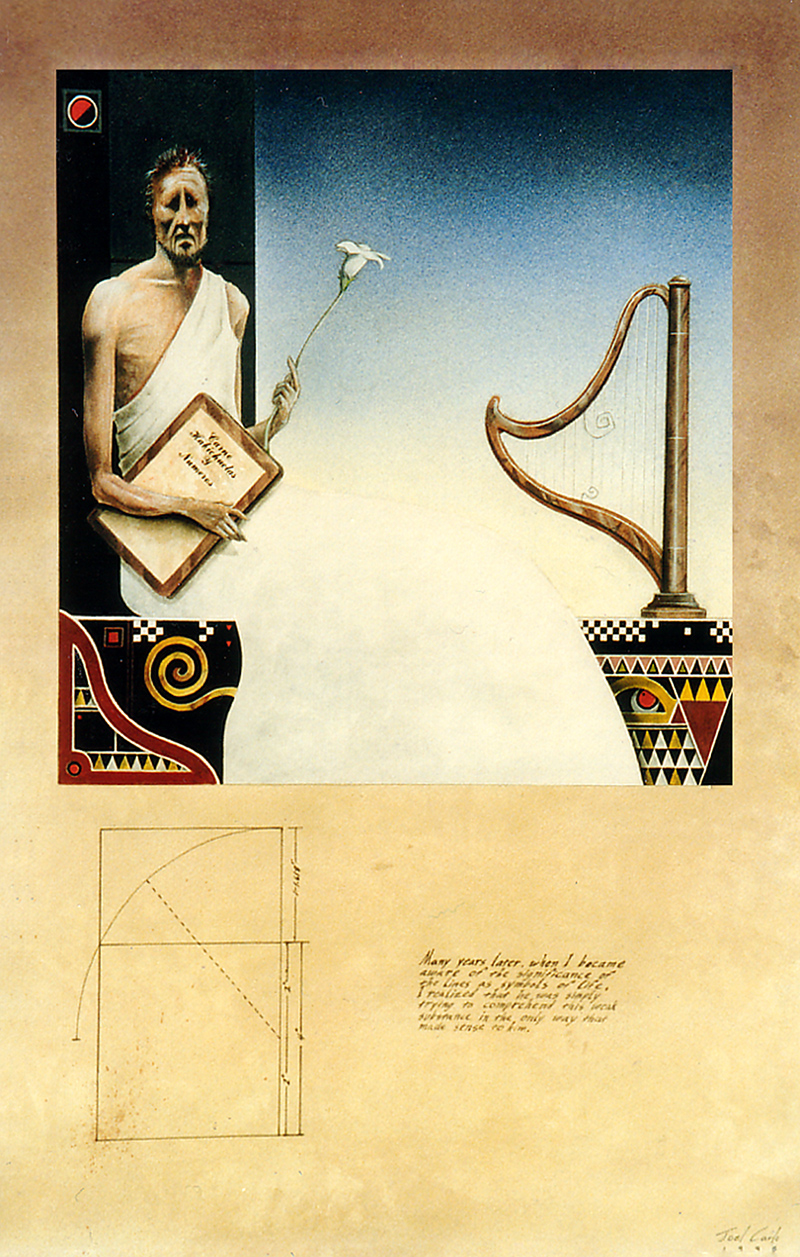### Project Description

Pythagoras is considered by some as history’s first pure mathematician and an extremely important figure in the development of mathematics. He is attributed for the Pythagorean Theorem, which claims that the sum of (the areas of) two small squares equals (the area of) the large one. In Pythagoras Explains, I used the theorem as an exercise in composition, while illustrating the famed mathematician as the primary theme within the piece. Acrylic on gouache paper, 1995.